# Introduction to Activation Functions in Neural Networks

In traditional ML approaches, the predicted Output from a parametric learning algorithm could be represented as Output = Weight.T * Input +Bias ( .T is used for transpose). This approach of representing Output in the form of weighted inputs was limiting ML developers from finding complex relationships present in the dataset. ML Researchers wanted machines to be more capable of finding patterns rather than only linear relations.

To make that possible, researchers passed the weighted inputs in a neural network from a non-linear function and called them "Activation functions". This boosted the capabilities of Neural Networks drastically and activated them to learn more complex patterns hidden in the dataset. Without these functions, an ANN was equivalent to the Linear Regression models, and in this blog, we will discuss details about them.

#### Key takeaways from this blog

After going through this blog, we will be able to understand the following things:

• What is an Activation function, and what will happen if we remove them from the ANN model?
• What are the properties of an Activation function?
• Popular linear and non-linear activation functions in ANN.

#### What is an Activation Function?

In ANN architectures, there are three types of layers: the Input, Hidden, and Output layers. All these layers are constituted of neurons used to connect layers. Neurons from the hidden and output layers take the weighted input from all the connections, add bias to it, and transform them with the help of a non-linear activation function. This transformed vector is the actual Output from a neuron.

These functions used to transform the weighted inputs are known as activation functions. It is called the "activation function" because these functions activate neurons to learn more complex non-linear patterns in the dataset. Sometimes, the Output of these functions becomes zero, and the neuron gets deactivated or does not participate in learning. This empowers activation functions to decide which neurons will participate, or in other words, get activated in the learning process.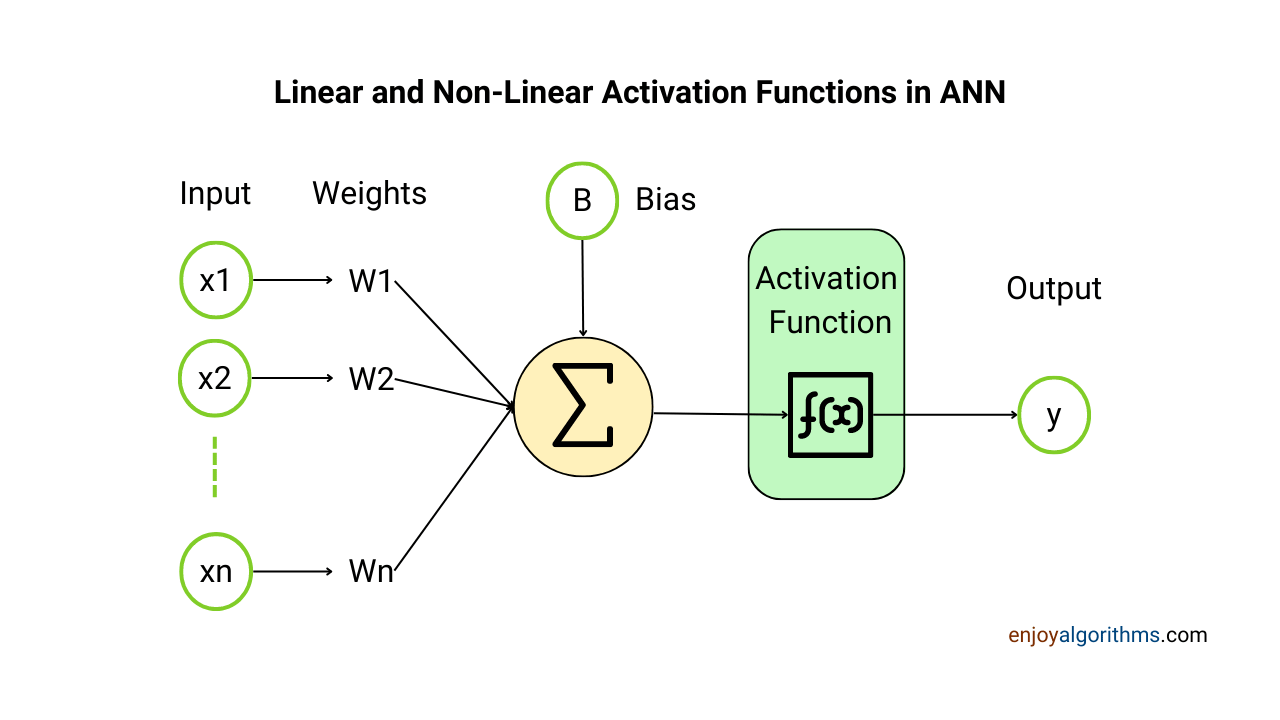Activation functions are also known as "transfer functions" in some literature. They are of mainly two types: Linear and Non-linear activation functions. However, most of these functions are non-linear, so some literature also refers to them as nonlinearity in the layer.

An activation function transforms the weighted input values, but one question should come to our mind: Any mathematical function could do this task. What's special about the activation function? How can we decide which function can become an activation function candidate? Let's explore.

#### What if we remove the Activation function from a Neural Network?

In a Neural Network, an activation function serves two major functionalities:

1. It makes our neural network capable of learning complex patterns hidden in the data by introducing nonlinearities. If we remove the activation function from a neural network, it will act like a linear (or polynomial) regression and fail to learn complex relationships among input and output samples. We will prove this shortly.
2. It clips the Output for any neuron and solves the problem of exploding or vanishing gradients. Vanishing and exploding gradients hamper the learning of the model as the gradient becomes too small and too large, respectively. If we remove the activation function, optimization algorithms like gradient descent might miss the minima of the cost function and fail to learn. We will discuss these things in detail later in this blog.

### What are the properties of an Activation function?

A function needs to follow some fundamental properties to become an activation function, and those are:

#### 1. Differentiability

In machine learning, weights and biases are initialized by random values, and then, as the training progresses, these values get tuned. During this tuning process, optimizers (like gradient descent) check the gradient values of the cost function with respect to various parameters. It is done to find whether the value of the parameters needs to be increased or decreased to achieve the minimum of the cost function.

For example, if we are solving any regression problem and using Mean Squared Error (MSE) as our cost function, then

``````## Cost will be

J(θ) = (1/n)Σ (Prediction - Actual) ^ 2``````

If we produce the Prediction from our neuron with randomly initialized weight values, it will look like this:

``````Prediction = activation(Weight*input + bias)

## Corresponding gradient of the cost function would be:

J'(θ)/δ(θ) = (1/n)Σ (δ(Prediction)/ δ(θ) - δ(Actual)/δ(θ)) ^ 2``````

Optimizers use the derivative of the cost function to find the best suitable weight and bias values for which cost becomes minimum. However, the activation function has also become a part of the cost function because it is present in the equation to find the predicted values. Hence, the activation function must also be differentiable for a smooth operation of derivatives.

Note: Theoretically, a function must always be differentiable, but in practice, this property is not hard and fast as we can have some alternative approach to get the derivatives. For example, there is one activation function ReLU with the mathematical formula of f(x) = max{0, X}. In theory, this function is not differentiable at x=0, but in practice, we forcefully define its derivative at x=0 while programming the ReLU activation function, turning it into a good candidate.

#### 2. Continuity

If any function is differentiable, it must be continuous, but vice versa is not valid. All continuous functions need not be differentiable always. As activation functions need to be differentiable, they should be continuous.

Note: This rule is also not hard-and-fast. There are some activation functions like the Binary/Step activation function f(x) = 0 if x < 0 and f(x) = 1 if x ≥ 0, which is a discontinuous function but still acts as an activation function because it helps in deciding which neurons should take part in learning.

#### 3. Boundedness

It is an important property for the activation function and dramatically influences the design of any neural network. If we select the wrong set of activation functions while designing the NN, the training process can suffer from either of the two problems:

An activation function typically needs to ensure the boundedness of the Output. For example, suppose its Output becomes very large. In that case, parameter updation will happen frequently (why?), and optimizers will fail to find the minimum value of the cost function. This problem is known as the exploding gradient problem, which affects the performance of the machine-learning model heavily. Hence, the Output for an activation function should be bounded by some defined range. In most cases, the Output of the activation function lies in the range of [0, 1] or [-1, 1].

Note 1: The ReLU activation function is an exception here. There is no upper bound on ReLU, and it is avoided if the weighted input values are very large.

Note 2: In the case of large output values from an activation function, the loss will be huge, and optimizers try to update the parameters frequently to ensure that the loss is going to a minimum. As these decisions will be frequent, the chances of missing the minimum point of the cost function will increase.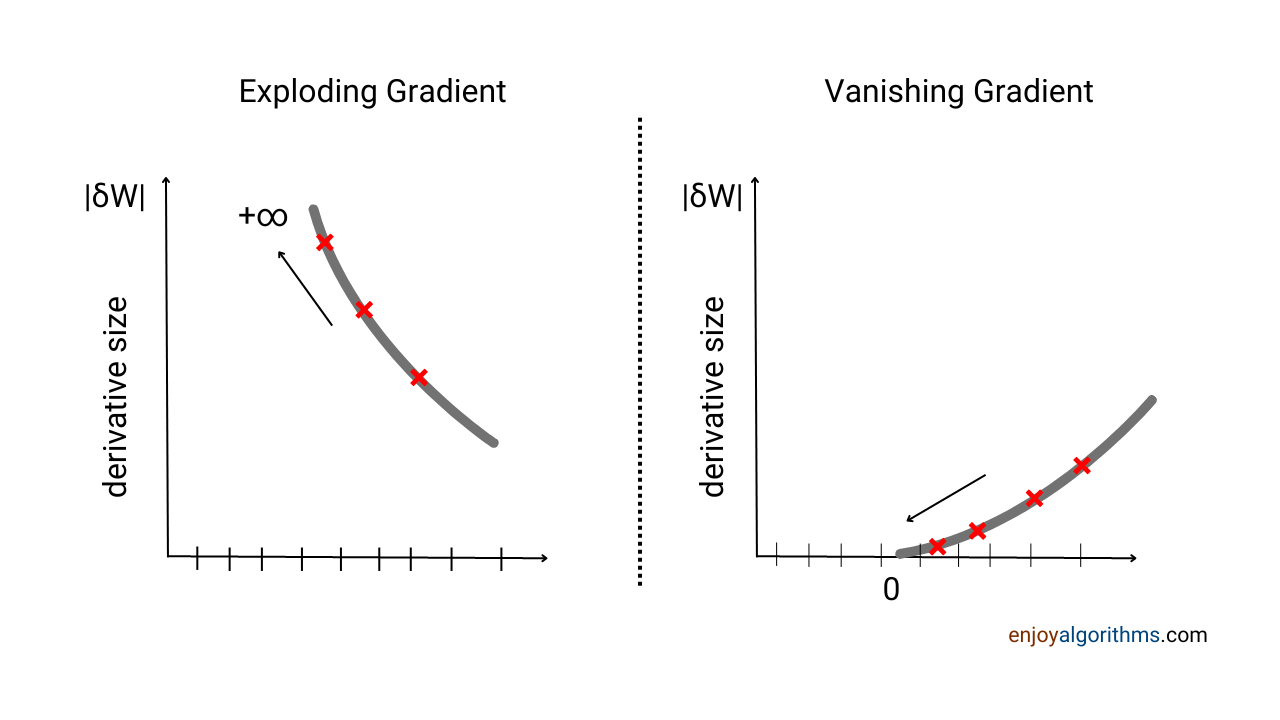Similarly, suppose the value for an activation function is too small. In that case, changes in the loss values will be insignificant as the activation function will be a part of the cost function. It will result in an infinite time to reach the minimum of the cost function. This problem is known as the vanishing gradient problem, where the gradient vanishes (tends to zero), resulting in optimizers fail to update parameters and, ultimately, the ML model fail to learn.

For example, suppose we have a two-layer neural network and activation functions corresponding to two hidden layers and one output layer are fh1 (x), fh2(x) and fo(x). If we consider all weights to be the identity matrix, then the Output will be equal to **fo(fh2(fh1(x))).** To update the parameters in the learning process, the optimization algorithms will calculate the gradient of this nested function, which will be fo' (fh2' (f_h1' (x))).

In most cases, the Output of the activation function is bounded in the range of [0, 1]; hence, the subsequent multiplication of values lesser than 1 will bring the gradient closer to zero. If the gradient diminishes to zero, the updation of parameters will not take place, and the model will fail to converge.

#### 4. The same function is used for all neurons in one layer

An activation function is defined once for the entire layer, and every neuron in that layer uses the same activation function. Also, in practice, all hidden layers contain the same activation function, but the activation functions for the hidden and output layers can be different.

For example, suppose there are 10 hidden layers, one input layer, and one output layer in the neural network. While designing this network, we need to define the activation function for each hidden layer individually and for the output layer. In practice, we use the same activation function for all hidden layers, but still, we define it individually for every layer.

#### 5. Activation of neurons

An activation function decides which neurons to activate during the learning process. If it produces an output equal to zero, that neuron will not participate in the learning process for that weighted input value. For example, a binary step function f(x) = 0 if x < 0 and f(x) = 1 if x ≥ 0 deactivates a neuron from the learning process if the weighted input value is lesser than 0.

#### 6. Computationally Cheaper

In the case of complex datasets like images and text, we stack huge amounts of hidden layers to learn even more complex relationships. An activation function is present in all neurons of these hidden layers and takes part in all the computations. For example, in some deep-learning models, the total number of parameters involved in learning is more than 10 Billion. We must pass all these parameters through the activation functions, which require very high processing power.

Hence, operations of activation functions need to be computationally cheaper. Otherwise, even huge processors will not be able to run a very simple model, which will decrease the accessibility of ANNs.

#### 7. Crossing origin

An activation function needs to cross the origin, which means the Output of the activation function should lie on both the positive and negative sides of the axis. It ensures the updation of parameters takes fewer iterations to find the minimum of the cost function. If an activation function's Output is always positive (or negative), it will take more epochs to train properly.

ReLU and sigmoid activation functions are exceptions here, which we will learn in the later part of this blog.

#### 8. Monotonic

It is conventionally said that the activation function must be monotonic. A monotonic function (or monotone function) represents a function that is either entirely non-increasing or non-decreasing.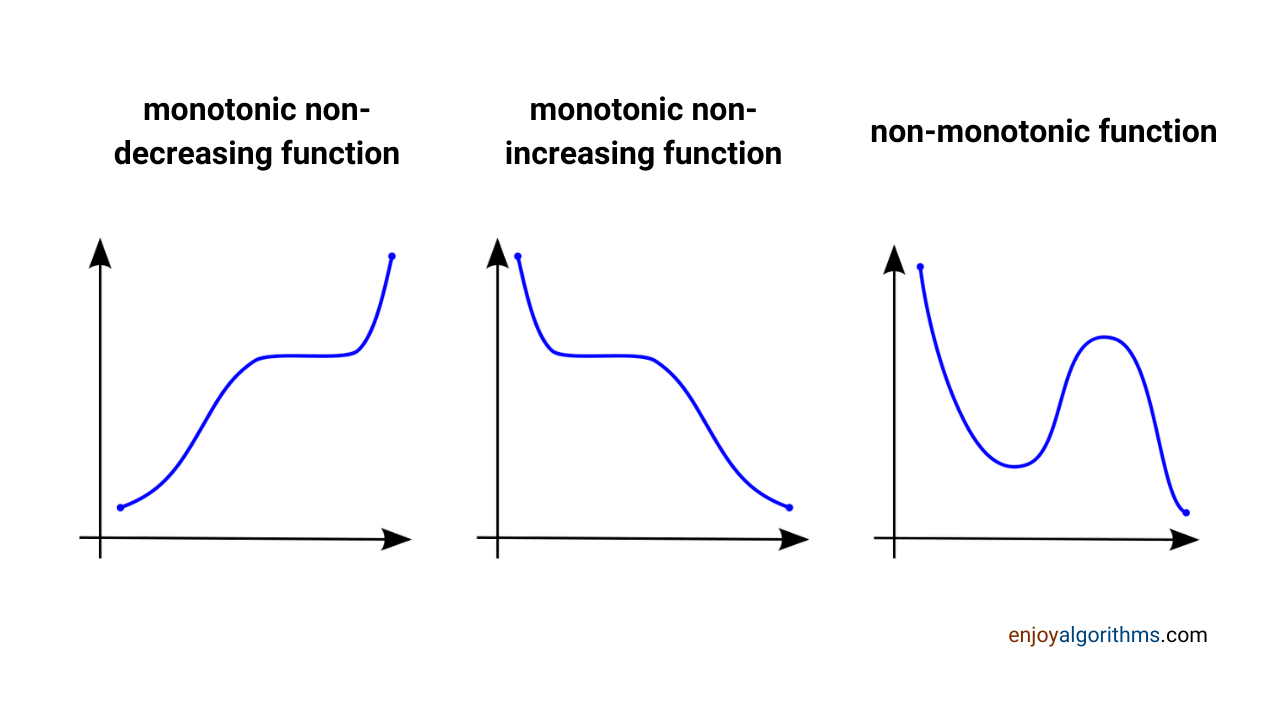An activation function that is not monotonic may activate the neurons for two drastically different input variables. This can create problems in case of updating parameters and achieving the minimum of the cost function.

For example, suppose for a weight value, a neuron gets deactivated, but we want that neuron to participate in learning. To do so, we can increase (or decrease) the weight values and bring neurons into the activation range. If the activation function is non-monotonic, we will not be sure whether an increase (or decrease) in weight will increase the Output from an activation function and activate it. This is one of the prime reasons we do not use Sine or Cosine functions as activation functions.

### Types of Activation Functions

From our mathematical understanding, a function can produce two Output types: Continuous and Discontinuous. We already have discussed that an activation function needs to be continuous except for the binary step activation function. Hence, almost all activation functions will be in the continuous category.

Now, as the Output is continuous, it can still be of two types: Linear or Non-linear. So let's learn about them in detail. But before that, see the basics of the binary step function.

### Binary Step Activation Functions

The binary step function is discontinuous but still acts as an activation function in neural networks. It is because of its fundamental properties, like computationally very cheap, monotonic, and has a tremendous ability to control the activity of any neuron.

Here, the output of the activation function is either 0 or 1, corresponding to whether any neuron will be active or not in any learning iteration. The Output of this activation function depends on a threshold value of the weighted input values. For example, if the weighted input value is greater than 0.6, the Output becomes 1 for that neuron, and this neuron becomes active; otherwise, the Output will become 0, and that neuron will not participate in learning.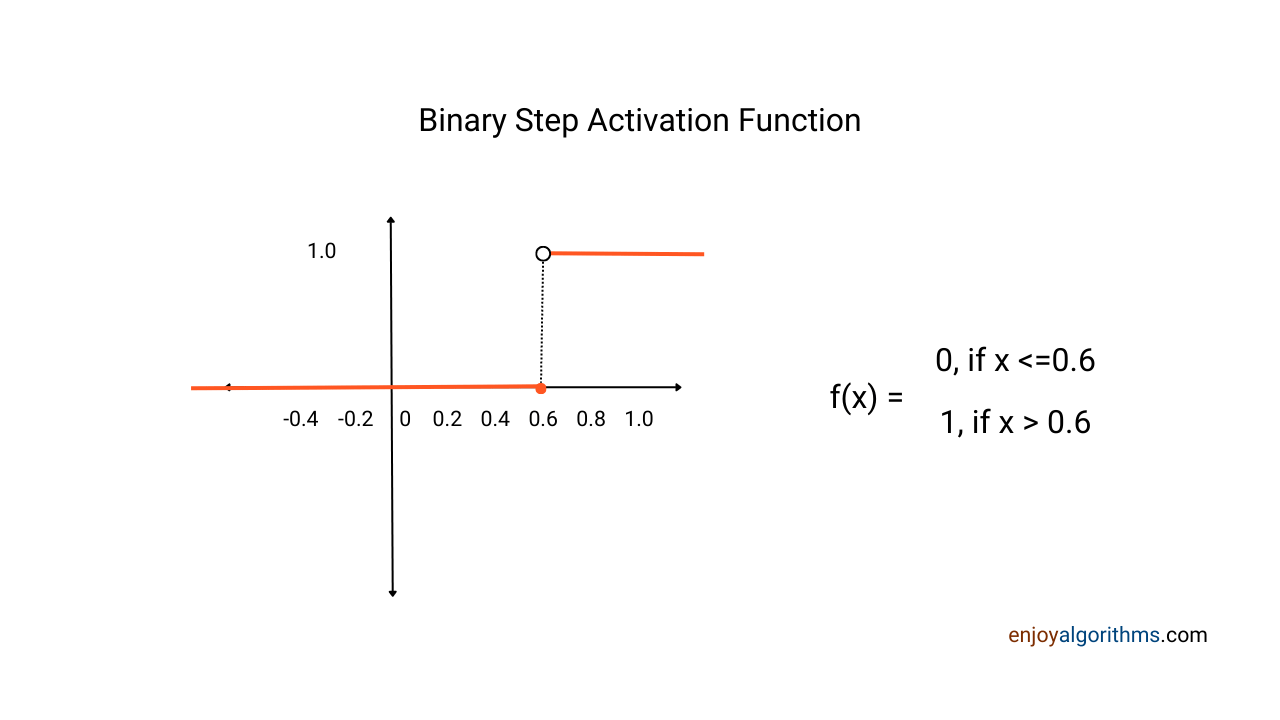### Linear Activation Functions

The linear activation function in neural networks represents the identity function, which means F(x) = x. It can be treated as no activation is applied to the weighted inputs.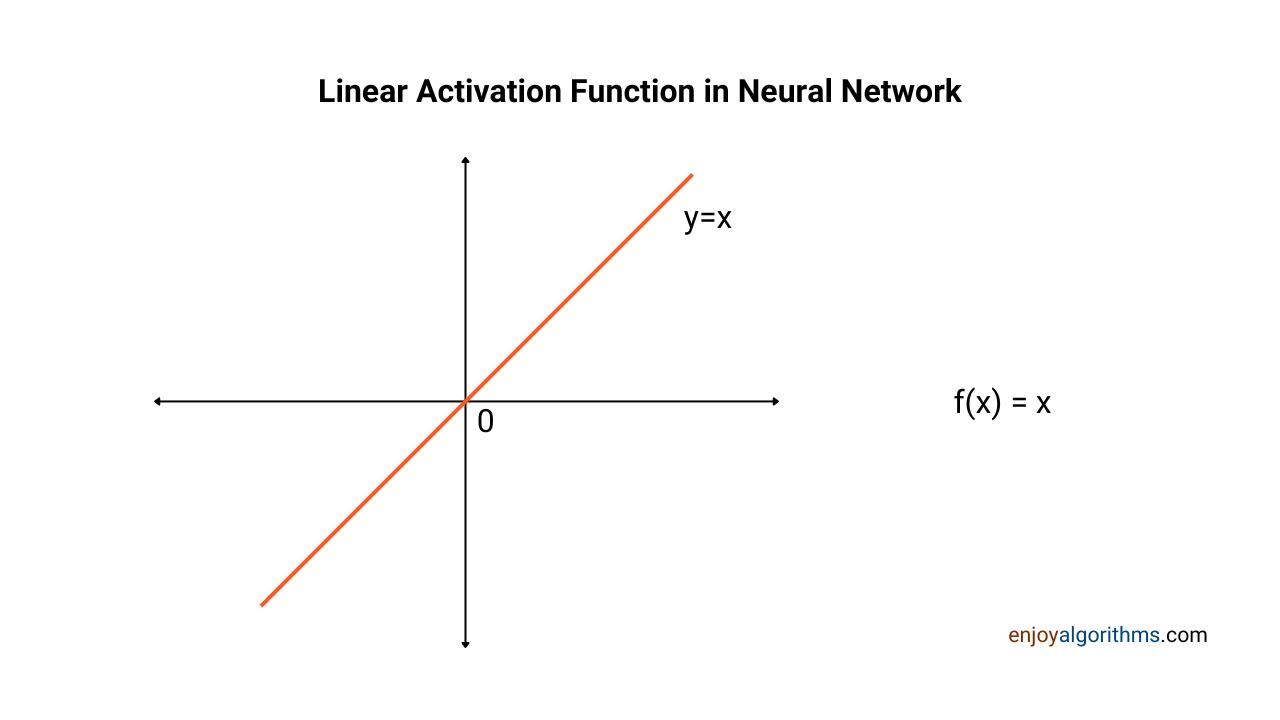We avoid using linear activation functions in the hidden layer of the Neural Networks for two primary reasons.

• It will activate every neuron, and there will be no control over the activation function to choose which neurons will be activated for better learning.
• All hidden layers of a neural network will collapse into a single hidden layer, and we will lose the capability to generate multiple-layer neural networks.

Let's understand these reasons mathematically. Suppose the input feature is x, and we have 2 hidden layers, each containing 2 nodes, in our neural network. Let's use the linear activation functions in both these layers and calculate the transformations.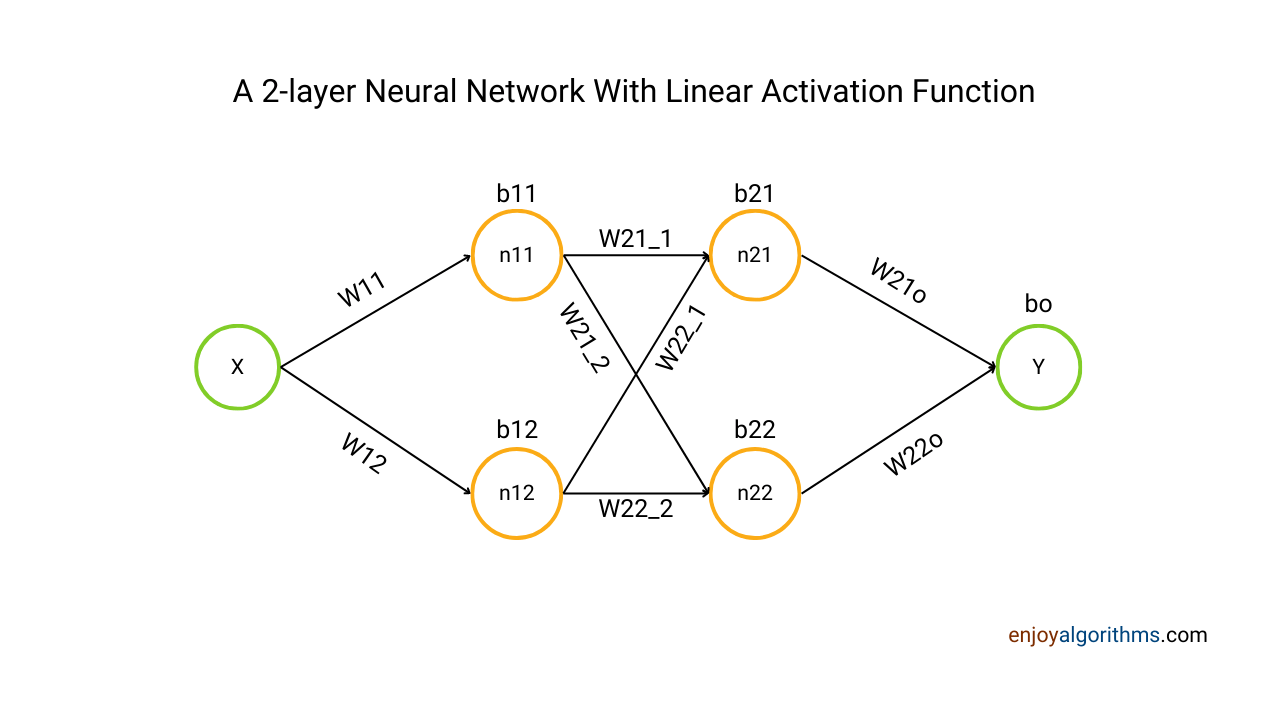``````Layer 1 activation function: f(x) = θ0 * x
Layer 2 activation function: f(x) = θ1 * x``````

Let's quickly define the notations used for weights and biases for all these connections and nodes.

``````W11 = Weight corresponding to connection between input node and first node of first hidden layer
W12 = Weight corresponding to connection between input node and second node of first hidden layer
b11 = Bias for first node in first hidden layer
b12 = Bias for second node in first hidden layer
Layer 1 activation function: f(x) = θ0 * x

W21_1= Weight corresponding to connection between first node in first hiiden layer and first node of second hidden layer
W22_1= Weight corresponding to connection between first node in first hiiden layer and second node of second hidden layer
W21_2= Weight corresponding to connection between second node in first hiiden layer and first node of second hidden layer
W22_2= Weight corresponding to connection between second node in first hiiden layer and second node of second hidden layer
b21 = Bias for first node in second hidden layer
b22 = Bias for second node in second hidden layer
Layer 2 activation function: f(x) = θ1 * x

W21o = Weight corresponding to connection between first node of second layer and the output node
W22o = Weight corresponding to connection between second node of second layer and the output node
bo = Bias for output node
Output node activation function: f(x) = θ2 * x``````

Now, let's perform the weight multiplication on the input vector x and then apply the activation function.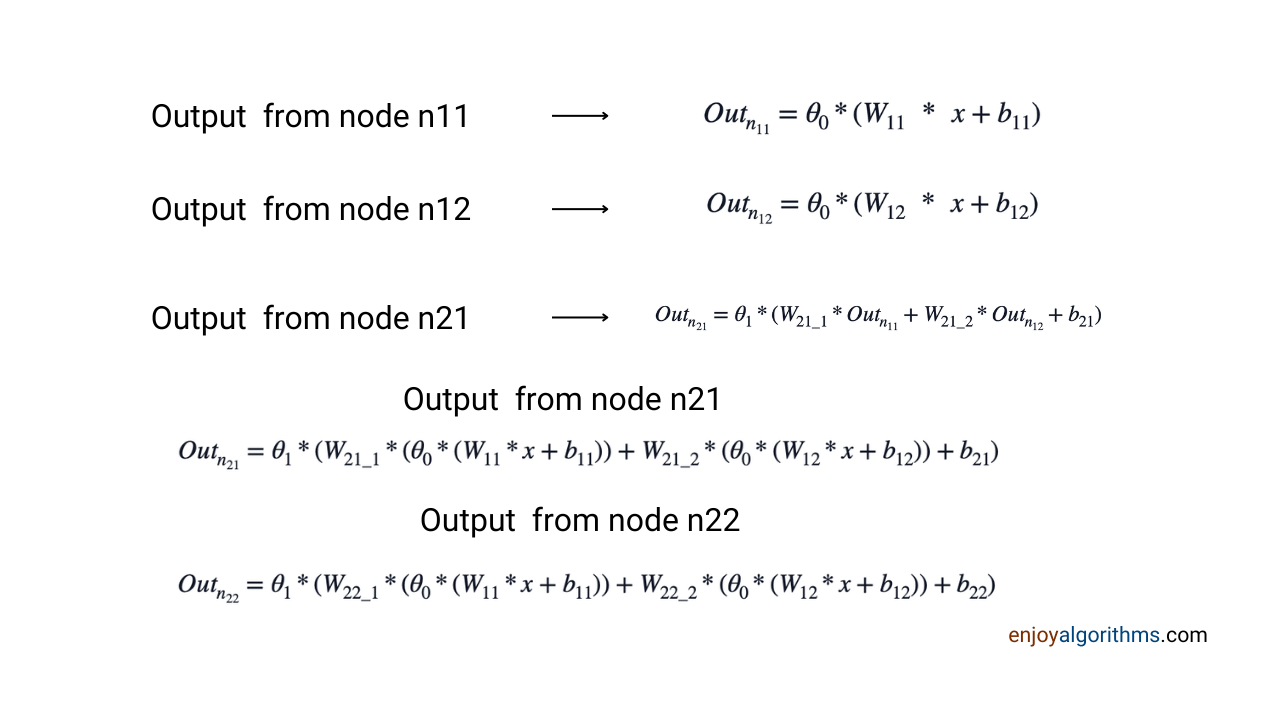Let's rearrange the output from the first node of the second layer (n21).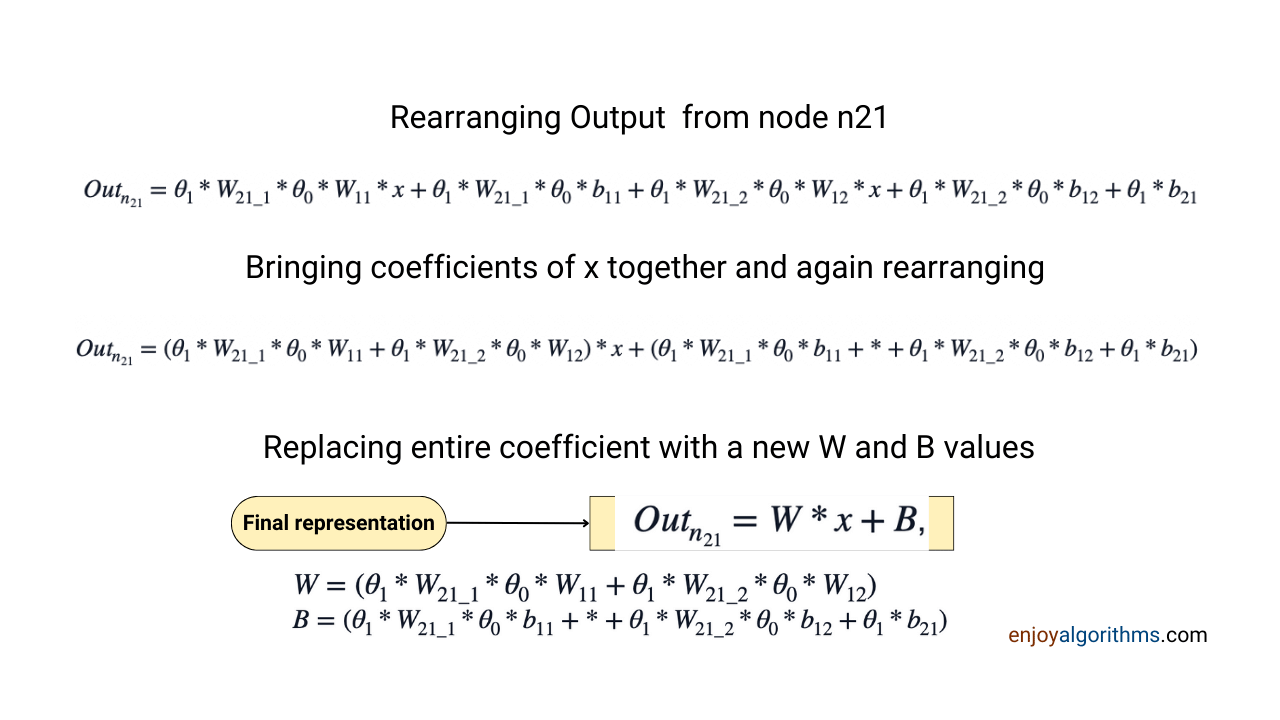If we observe, the entire Output from the n21 node can be represented in terms of updated weight and bias values. Suppose we keep rearranging the outputs till the output node. In that case, the final representation will result in a simple linear regression form, i.e., Y = W * X + B, which can be represented through a single layer.

So, the multiple-layer neural network collapsed into a single-layer network, and the entire neural network algorithm started seeming like a linear regression model. Hence, we avoid using linear activation functions in the hidden layers.

### Non-Linear Activation Functions

A non-linear activation function is the non-linear transformation of weighted inputs received at the nodes. It is the prime reason for the success of Neural networks in providing solutions to problems across multiple domains. These functions gave flexibility in two areas:

• While finding the minima of the cost function, the optimization algorithm calculates the derivates of the cost function with respect to all the parameters involved. As non-linear activation functions are differentiable, they allow this operation and also suggest which neurons can contribute most to the learning process.
• It allows us to stack multiple layers and learn more complex patterns hidden in the data. Even if the problem is too complex to be learned by traditional approaches, we can increase the number of hidden layers to extract the maximum information in the data.

During the 1970s, ML researchers were struggling to make Neural Networks learn XOR gate properties. After a decade of struggle, scientists discovered the multi-layer perceptron to make it learn to XOR gate, and we have covered this in our designing perceptron blog.

There are many non-linear activation functions present these days in the literature. But our focus here will be to briefly cover the four most used non-linear activation functions in the literature on Machine Learning and Neural Networks.

Note: In our subsequent blogs, we will cover codes for these activation functions and tips on when to use which activation function while designing our neural network.

#### 1. Sigmoid or Logistic Function:

The logistic function, popularly known as the Sigmoid function, is one of the most widely used activation functions in Neural Networks applications. The mathematical formula for the sigmoid activation function is: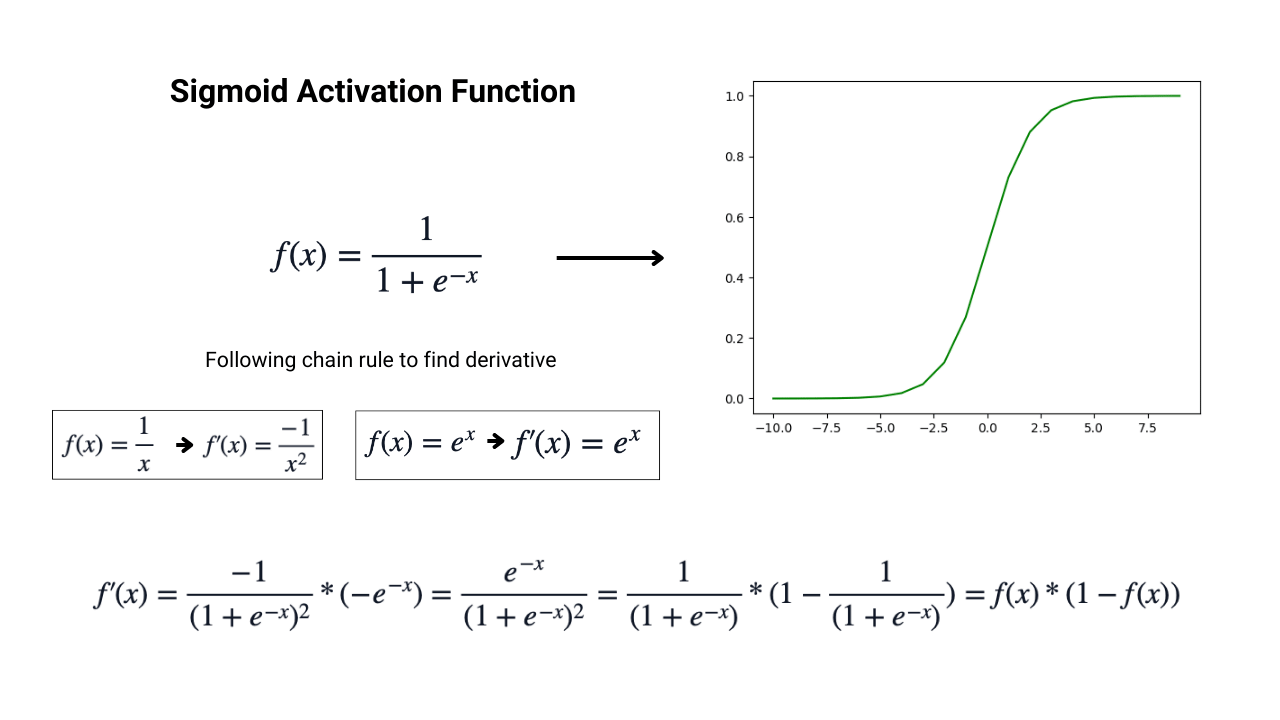The sigmoid activation function is continuous, differentiable, monotonic, and bounded in the range of (0, 1). But as the exponential calculation is expansive, Sigmoid is also computationally expensive. To compensate for this, the gradient calculation for this activation function is straightforward, which gives it a tradeoff. In the image above, we can see the calculation of the gradient for the sigmoid function, which is nothing but f(x) * (1- f(x)).

The Output of the sigmoid function is confined in the range of (0,1), so when we find the derivative of the Output, it becomes very small as we multiply two values with a magnitude less than 1. This makes the sigmoid activation function suffer from vanishing gradient issues. Also, as this function is not crossing the origin, we need more epochs to reach better results.

To solve these hurdles of the Sigmoid activation function, tanh or hyperbolic tangent activation function was introduced.

#### 2. Tanh or Hyperbolic Tangent Activation Function:

The hyperbolic tangent action function, popularly known as the tanh activation function, is slightly more popular than the sigmoid activation function. The mathematical formula and corresponding graph for the tanh activation function are: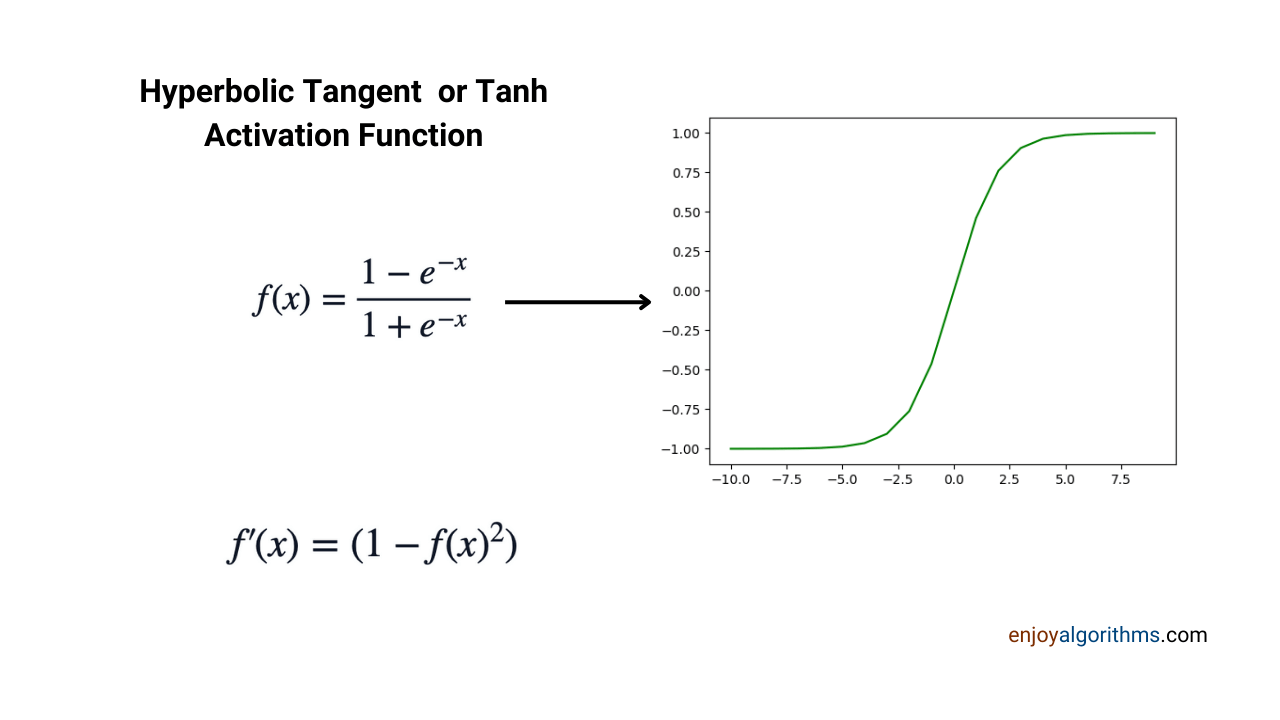If we look carefully, the graphs of tanh and Sigmoid look similar; the only difference is that tanh is crossing the origin. So, the tanh activation function is also continuous, differentiable, monotonic, and bounded in the range of (-1, 1). For the same reason as in Sigmoid, exponential calculation is involved, making it computationally expansive. However, the gradient calculation is simple: f' (x) = 1-f(x)².

If we arrange the mathematical formula for tanh, we will find that tanh (x) = 2*sigmoid (2x) — 1. So tanh contains all the properties of Sigmoid with the additional benefit of zero crossing. Hence, it provides better results while building neural network applications.

#### 3. Rectified Linear Unit (ReLU) Activation Function:

The rectified linear unit, popularly known as ReLU, has become the most popular activation function and the first choice in many applications. It was first introduced in 1975 but was adopted very late in 2010 after publishing the research paper of Nair & Hinton

The mathematical formula and corresponding graph for the ReLU activation function are: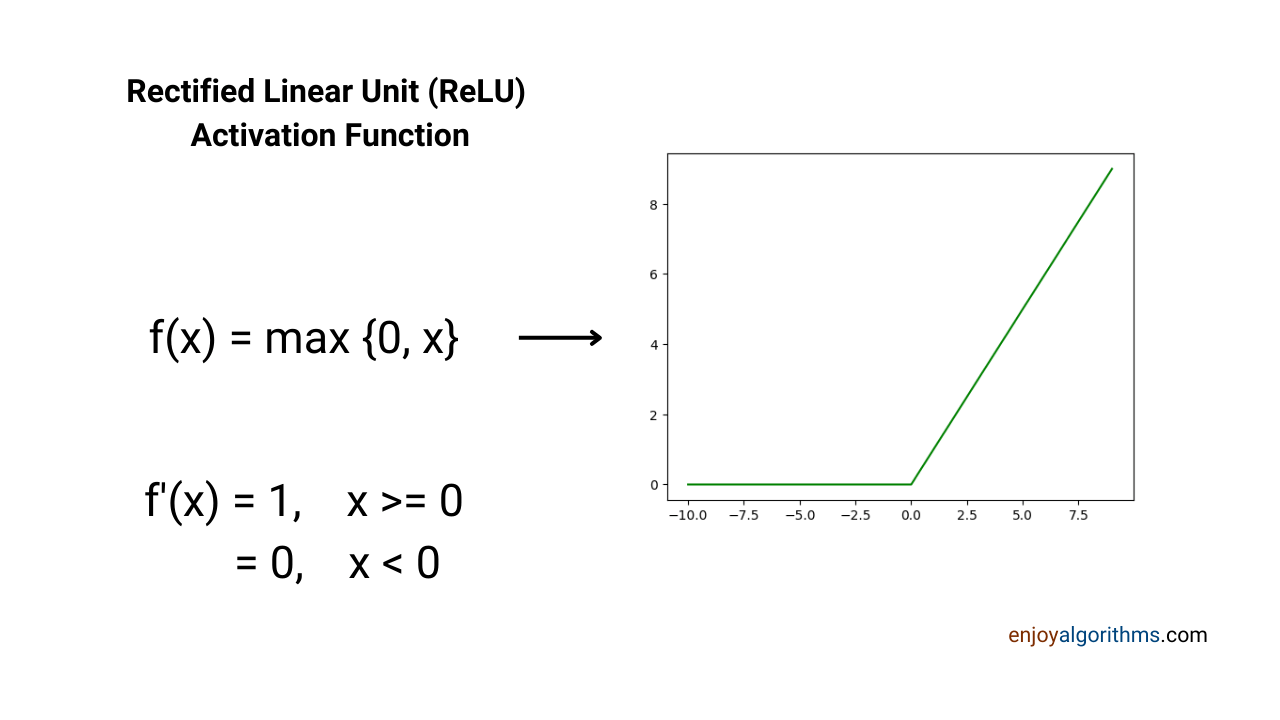It is continuous, monotonic, and computationally very cheap. But it is not differentiable everywhere. If we recall the differentiability theory, the above function is not differentiable at x = 0. But in practice, this is not a problem, and we can assume the gradient of the relu activation function at x=0 as 0. This creates no problem and gives too many benefits over tanh and sigmoid activation functions.

The computations involved in this function are very cheap, and based on experiments, researchers have proved that ReLU is six times faster to train when compared to tanh or Sigmoid. Also, as the Output is not bounded here when x is positive, ReLU never faces vanishing gradient problems.

#### 4. Softmax Activation Function

The softmax activation function is popular and used only in the output layer when solving multi-class classification problems. The mathematical formula for this is:

``f(x) = exp(xi) / Σ exp(xi)``

This function produces a vector of values that sum to 1. For example, if there are three classes and we are solving a multi-class classification problem, it will give a vector like [0.6, 0.3, 0.1]. The sum of this vector is 1.0, and the individual entities of the vector can represent the probabilities of corresponding classes. In the above example, class 1 likely has a probability of 60% to be predicted, class 2 has 30%, and class 3 has 10%.

#### Popular Interview Questions on Activation Functions

Activation function is one of the most important topics in deep learning or neural networks. The change of activation function in one layer can drastically change the learning behavior of our model. Interviewers love to check the candidate's knowledge on this topic, and some popular questions that can be asked are:

1. What is an Activation function? Which activation function you used to solve the problem statement you mentioned in your resume?
2. What will happen if we remove the activation function from a neural network?
3. Why do we not use linear activation functions in hidden neural network layers?
4. Sine or Cosine functions are bounded, but why can't we use these functions as an activation function?
5. What are the problems of the Sigmoid activation function?
6. If ReLU is not always differentiable, how can it become an activation function?
7. Explain the Vanishing and Exploding gradient problem in ANNs.
8. Why does ReLU not suffer a vanishing gradient problem?

### Conclusion

Activation function is one of the most prominent discoveries in Machine Learning, as it allows learning complex patterns. It is one of the most asked topics in ML interviews and, hence, a vital topic to be familiar with. In this article, we have discussed the basics of Activation functions and their importance in designing ANNs. We have covered its properties, types, and popular variations in the market and hope you learned something new.

Enjoy Learning!

☆ 16-Week Live DSA Course
☆ 10-Week Live DSA Course